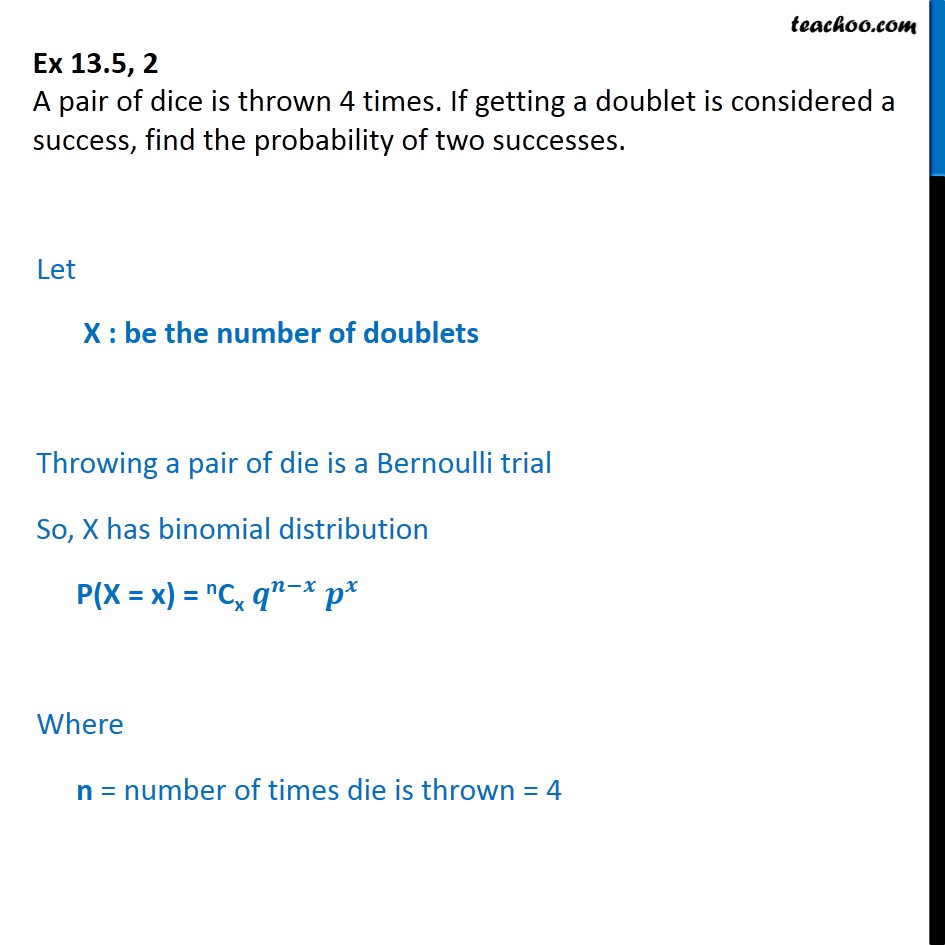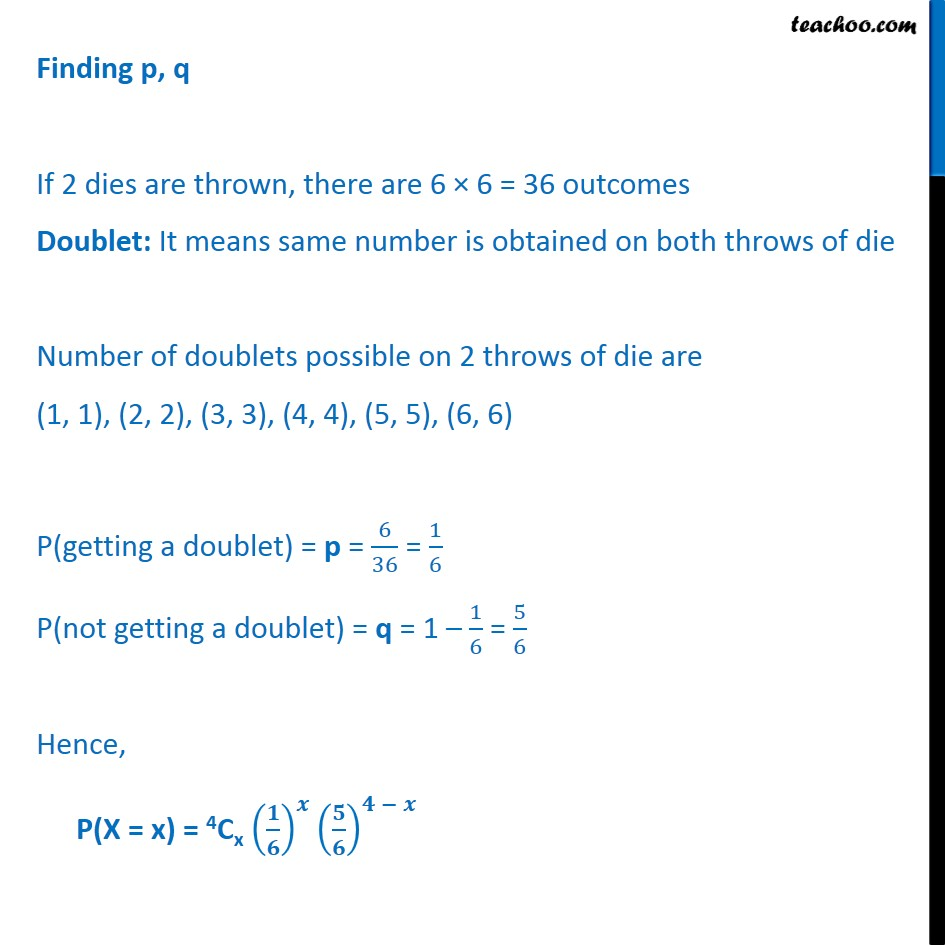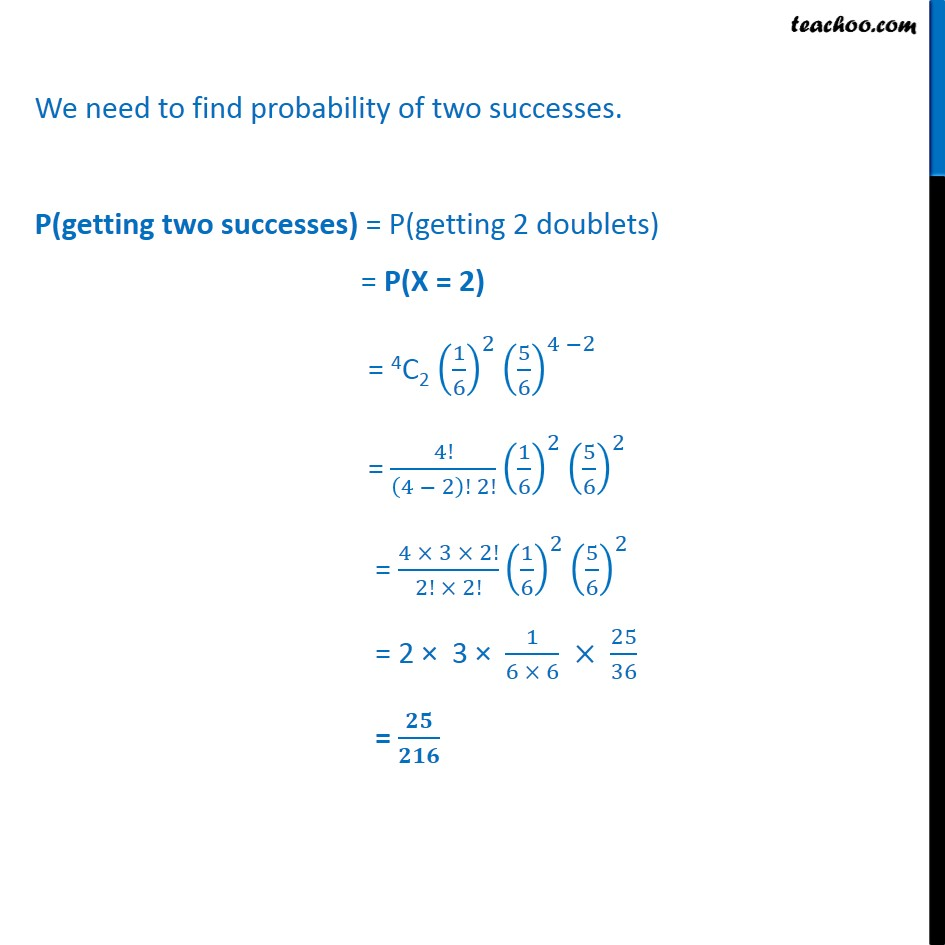Bernoulli Trial

Chapter 13 Class 12 Probability
Serial order wiseLearn in your speed, with individual attention - Teachoo Maths 1-on-1 Class

### Transcript

Question 2 A pair of dice is thrown 4 times. If getting a doublet is considered a success, find the probability of two successes. Let X : be the number of doublets Throwing a pair of die is a Bernoulli trial So, X has binomial distribution P(X = x) = nCx 𝒒﷮𝒏−𝒙﷯ 𝒑﷮𝒙﷯ Where n = number of times die is thrown = 4 Finding p, q If 2 dies are thrown, there are 6 × 6 = 36 outcomes Doublet: It means same number is obtained on both throws of die Number of doublets possible on 2 throws of die are (1, 1), (2, 2), (3, 3), (4, 4), (5, 5), (6, 6) P(getting a doublet) = p = 6﷮36﷯ = 1﷮6﷯ P(not getting a doublet) = q = 1 – 1﷮6﷯ = 5﷮6﷯ Hence, P(X = x) = 4Cx 𝟏﷮𝟔﷯﷯﷮𝒙﷯ 𝟓﷮𝟔﷯﷯﷮𝟒 − 𝒙﷯ We need to find probability of two successes. P(getting two successes) = P(getting 2 doublets) = P(X = 2) = 4C2 1﷮6﷯﷯﷮2﷯ 5﷮6﷯﷯﷮4 −2﷯ = 4!﷮ 4 − 2﷯! 2!﷯ 1﷮6﷯﷯﷮2﷯ 5﷮6﷯﷯﷮2﷯ = 4 × 3 × 2!﷮2! × 2!﷯ 1﷮6﷯﷯﷮2﷯ 5﷮6﷯﷯﷮2﷯ = 2 × 3 × 1﷮6 × 6﷯ × 25﷮36﷯ = 𝟐𝟓﷮𝟐𝟏𝟔﷯